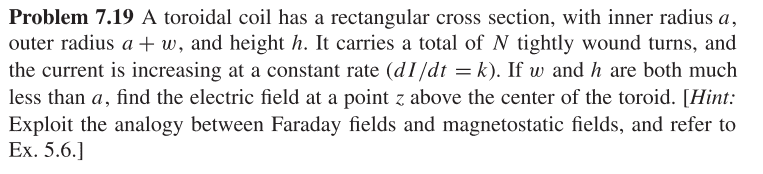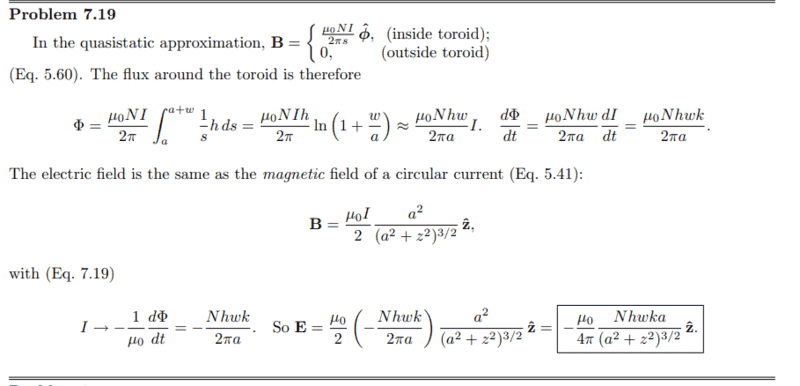# Going From EMF to Faraday's Law to find E-field in my HW problem

• ChanceLiterature
In summary, Griffiths explains how to find E field in a magnetic field above a current loop by solving for B. He then says that this B field is equivalent to the magnetic field above a ring with current I. However, he can't explicitly explain how this is the case and I couldn't find any mathematical justification of there equivalence.

#### ChanceLiterature

Homework Statement
Ok, so this out of Giffth's intro to Edynamics. Problem 7.19:
A toroidal coil has a rectangular cross section with inner radius a, outer radius a+w and height h. It has N tightly wound loops. dI/dt= k. Additionally, w, h << a. Find E above center of toroid at height z.
Relevant Equations
B inside toroid mu NI/ 2 /PI / s; s being from cylindrical.
B outside = 0
Ok, so I understand how to find dphi/dt that is integral of -d/dt(B "dot" da). In this case I find a Phi that is a constant in space in time which causes me confusion in next step.
Edit: dphi/dt is constant...

Grithff's then says E field same as a Mag field above center of circular current. He writes the B found from solving Biot-Savart for a ring with current I, and says that in this senecio I is equal to -1/mu dPhi/dt.

Firstly, how the heck am I supposed to know to make this substitution based on the textbook?

Secondly, how does this make sense. How can I take a statement about EMF and faradays law that will gives me E dot dl of a closed loop and use that to find the E at any point in space. Especially, when the EMF is not a function of time or space!

I understand the idea that E field in this problem is equivalent to the B field in the prior problem. However, I can't explicitly understand how they are the same and I couldn't find any mathematical justification of there equivalence.

I would appreciate any help anyone can give me. This problem has frustrated me to no end. Therefore, I apologize for any moodiness while writing the problem.

Last edited:
Problem:Solution from solution manual:ChanceLiterature said:
Homework Statement:: Ok, so this out of Giffth's intro to Edynamics. Problem 7.19:
A toroidal coil has a rectangular cross section with inner radius a, outer radius a+w and height h. It has N tightly wound loops. dI/dt= k. Additionally, w, h << a. Find E above center of toroid at height z.
Relevant Equations:: B inside toroid mu NI/ 2 /PI / s; s being from cylindrical.
B outside = 0

Ok, so I understand how to find dphi/dt that is integral of -d/dt(B "dot" da). In this case I find a Phi that is a constant in space in time which causes me confusion in next step.
The current ##I## is changing with time. How can the flux be constant?

ChanceLiterature said:
Grithff's then says E field same as a Mag field above center of circular current. He writes the B found from solving Biot-Savart for a ring with current I, and says that in this senecio I is equal to -1/mu dPhi/dt.

Firstly, how the heck am I supposed to know to make this substitution based on the textbook.

Secondly, how does this make sense. How can I take a statement about EMF and faradays law that will gives me E dot dl of a closed loop and use that to find the E at any point in space. Especially, when the EMF is not a function of time or space!

I understand the idea that E field in this problem is equivalent to the B field in the prior problem. However, I can't explicitly understand how they are the same and I couldn't find any mathematical justification of there equivalence.
He explains all of this pretty clearly at the beginning of that section 7.2.2 (in the fourth edition). If ##\mathbf{E}## is a purely induced field, it satisfies the same equations as a magnetostatic field with ##\mu_0 \mathbf{J}## replaced by the ##-\partial_t \mathbf{B}##.

•Delta2 and ChanceLiterature
Apologizes, I meant to say dphi/dt is constant in time and space.
Ok, I will look over that section in more detail now.

vela said:
The current ##I## is changing with time. How can the flux be constant?

He explains all of this pretty clearly at the beginning of that section 7.2.2 (in the fourth edition). If ##\mathbf{E}## is a purely induced field, it satisfies the same equations as a magnetostatic field with ##\mu_0 \mathbf{J}## replaced by the ##-\partial_t \mathbf{B}##.
Wow! I have no idea how I missed that! That raises a different question though. If we have EQ 7.18 from section 7.2.2, then why in the solutions does Griffiths find phi first when EQ 7.18 only requires partial of B. Do I need to find phi?

The ##\partial_t B## is like the current density ##J##, but the magnetic field due to the loop depends on the current ##I##, which is analogous to the magnetic flux ##\Phi_B##.

•ChanceLiterature and Delta2
Thank you!